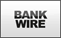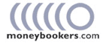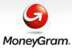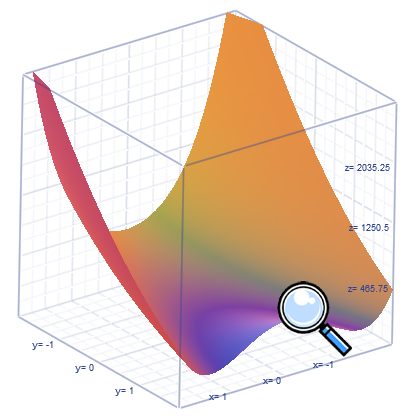﻿ InnerSoft STATS - Statistics and Financial App

# InnerSoft STATS

A Statistics and Financial Application## Introducing InnerSoft

InnerSoft is a company specialized in developing applications for maths, science and CAD. We develop tools to automate and reduce time consuming tasks in scientific, architecture and engineering consulting firms. Let us know your ideas to improve the productivity of your company, and we will try to provide a solution saving you hours of work.

InnerSoft STATS is an application to compute statistics for parameter estimation. Using Demo Version, you will be able to compute statistics with no restrictions.Price is 30 dollars. Using the full version, you will be able to open and save files, and export outputs to Excel. You can pay by PayPal.You can also pay (including any fees with your payment) by:
 Bank-to-bank wire transfer Western UnionMoneybookers MoneyGram## Contact

For further information, contact by email at innersoft@itspanish.org

You can also call us:## Available Statistical Methods

Frequency Tables: Frequency, Percent, Cumulative Frequency, Cumulative Percent, Valid Percent, Valid Cumulative Percent Descriptive Statistics: Mean, Variance, Standard deviation, Coefficient of variation, Quartiles, Percentiles, Skewness, Kurtosis, Mode, Interquartile range, Sum of Squares.
Pearson Chi-Square Test, Yates's Continuity Correction, Likelihood Ratio G-Test, Mantel-Haenszel Chi-Square Test, One sided and two sided Fisher’s Exact Test, McNemar asymptotic, Edwards Continuity Correction, McNemar Exact Binomial, Mid-P McNemar Test, McNemar-Bowker Test, Odds Ratio, Relative Risk, Attributable risk, Relative Attributable Risk, Number Needed to Harm, Attributable Risk per Unit, Etiologic Fraction, Cohen's Kappa Test. Phi Coefficient, Contingency Coefficient, Standardized Contingency Coefficient, Cramer's V, Tschuprow's T, Symmetric Lambda, Asymmetric Lambda, Symmetric Uncertainty Coefficient, Asymmetric Uncertainty Coefficient, Gamma, Sommers’ d, Kendall’s tau-b, Kendall’s tau-c.
One-Sample Test: One-sample z-test, One-sample t-test, Chi-squared test for variance.
Two-Sample Test: Student's t-test for Independent samples (pooled t-test for equal variances and unpooled t-test for unequal variances), Student's t-test for Paired samples, Two-sample F-test of equality of variances.
One-Way ANOVA with multiple comparisons methods: Scheffe, Tukey HSD, Sidak, Fisher LSD, Bonferroni. Welch’s Test for equality of means, Brown–Forsythe Test for equality of means
Homoscedasticity Test: Levene's Test, Brown–Forsythe Test for equality of variances, Bartlett's Test
Bivariate Correlation Tests: Matrix of covariances, Pearson Product-Moment Correlation Coefficients, Kendall's Tau-b Correlation Coefficients, Spearman’s Correlation Coefficients
Parametric Value at Risk by the Variance-Covariance Method for single assets and portfolios. Marginal Value at Risk, Component Value at Risk, Incremental Value at Risk, Conditional Value at Risk, Expected Shortfall, Expected Tail Loss or Average Value at Risk.
Exponentially Weighted Moving Average (EWMA) Forecast
Financial Formulas: Accumulation Distribution, Average True Range, Bollinger Bands, Chaikin Oscillator, Commodity Channel Index, Detrended Price Oscillator, Ease of Movement, Envelopes, Forecasting, Mass Index, Money Flow, Moving Average Convergence/Divergence, Exponential Moving Average, Simple Moving Average, Triangular Moving Average, Triple Exponential Moving Average, Weighted Moving Average, Negative Volume Index, On Balance Volume, Performance, Positive Volume Index, Median Price, Typical Price, Weighted Close, Price Volume Trend, Rate of Change Relative Strength Index, Standard Deviation, Stochastic Indicator, Volatility Chaikins, Volume Oscillator, William's %R
Linear Regression: Unstandardized Predicted Values, Prediction Intervals for Mean, Prediction Intervals for Individual, Standardized Predicted Values, Mahalanobis Distances, Cook's Distances, Centered Leverage values, Unstandardized Residuals, Standardized Residuals, Studentized Residuals, Durbin-Watson statistics.
Curve Estimation: Linear, Quadratic, Cubic, Quartic, Quintic, Sextic, Inverse, Logarithmic, Power, Exponential, Compound, S-curve, Logistic, Growth.
Create Time Series: Difference, Seasonal difference, Centered moving average, Prior moving average, Running medians, Cumulative sum, Lag, Lead.
Univariate GARCH(1,1): Conditional Variance. Conditional Volatility. Predicted Volatility.

## Available Graphs

Bar chart type, Bubble chart type, Candlestick chart type, Column chart type, Doughnut chart type, Fast Line chart type, Fast Point chart type, Funnel chart type, Kagi chart type, Line chart type, Pie chart type, Point chart type, Point And Figure chart type, Pyramid chart type, Radar chart type, Range chart type, Range Bar chart type, Range column chart type, Renko chart type, Spline chart type, Spline area chart type, Spline range chart type, Stacked area chart type, Hundred-percent stacked area chart type, Stacked bar chart type, Hundred-percent stacked bar chart type, Stacked column chart type, Hundred-percent stacked column chart type, StepLine chart type, Stock chart type, Three Line Break chart type.

## Video Tutorials

Bivariate Correlation in InnerSoft STATS

Multiple and Simple Linear Regression in INNERSOFT STATS

Curve Estimation with InnerSoft STATS

How to fit a GARCH(1, 1) Model in InnerSoft STATS

How to use Financial Formulas - INNERSOFT STATS

How to perform One-Way ANOVA - INNERSOFT STATS

Charts for Two Categorical Variables - INNERSOFT STATS

How to draw Candlestick, Point And Figure, Stock chart - INNERSOFT STATS

How to Draw Renko, Kagi and Three Line Break charts - INNERSOFT STATS

## Open Source Dependencies